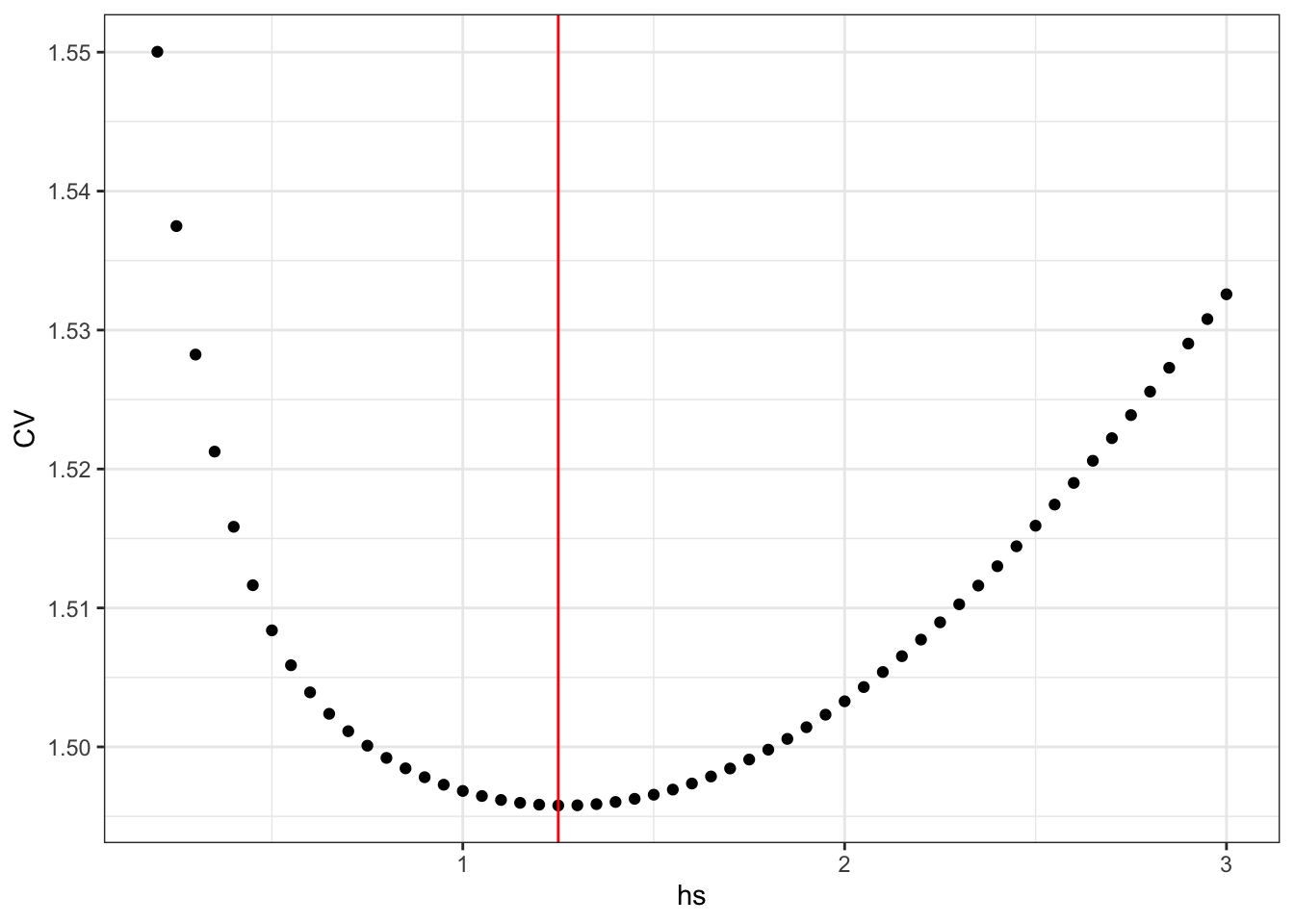## 3.2 Kernel methods

### 3.2.1 Nadaraya–Watson kernel smoothing

The section before introduced the basic idea of nearest neighbor smoothing using a fixed number of neighbors. A very similar idea is to use a fixed neighborhood, $$B_i$$, say, around $$x_i$$. This leads to the estimator $\hat{f}_i = \frac{\sum_{j=1}^n y_j 1_{B_i}(x_j)}{\sum_{j=1}^n 1_{B_i}(x_j)},$ which is simply the average of the $$y$$-s for which the corresponding $$x$$-s fall in the neighborhood $$B_i$$ of $$x_i$$. Note that contrary to the nearest neighbor estimator, the denominator now also depends on the $$x$$-s.

In a metric space the natural choice of $$B_i$$ is the ball, $$B(x_i, h)$$, around $$x_i$$ with some radius $$h$$. In $$\mathbb{R}$$ with the usual metric (or even $$\mathbb{R}^p$$ equipped with any norm-induced metric) we have that
$1_{B(x_i, h)}(x) = 1_{B(0, 1)}\left(\frac{x - x_i}{h}\right),$ thus since $$B(0, 1) = [-1, 1]$$ in $$\mathbb{R}$$ $\hat{f}_i = \frac{\sum_{j=1}^n y_j 1_{[-1, 1]}\left(\frac{x_j - x_i}{h}\right)}{\sum_{l=1}^n 1_{[-1, 1]}\left(\frac{x_l - x_i}{h}\right)}.$ This nonparametric estimator of the conditional expectation $$E(Y \mid X = x_i)$$ is closely related to the kernel density estimator with the rectangular kernel, see Section 2.2, and just as for this estimator there is a natural generalization allowing for arbitrary kernels instead of the indicator function $$1_{[-1, 1]}$$.

With $$K : \mathbb{R} \mapsto \mathbb{R}$$ a fixed kernel the corresponding kernel smoother with bandwidth $$h$$ becomes $\hat{f}_i = \frac{\sum_{j=1}^n y_j K\left(\frac{x_j - x_i}{h}\right)}{\sum_{l=1}^n K\left(\frac{x_l - x_i}{h}\right)}.$ This smoother is known as the Nadaraya–Watson kernel smoother or Nadaraya–Watson estimator. See Exercise 3.1 for an additional perspective based on bivariate kernel density estimation.

The Nadaraya–Watson kernel smoother can be implemented in much the same way as the kernel density estimator, and the run time will inevitably scale like $$O(n^2)$$ unless we exploit special properties of the kernel or use approximation techniques such as binning. In this section we will focus on implementing bandwidth selection rather than the kernel smoother itself. The basic kernel smoothing computation is also implemented in the ksmooth function from the stats package.

f_hat <- ksmooth(
Nuuk_year$Year, Nuuk_year$Temperature,
kernel = "normal",
bandwidth = 10,
x.points = Nuuk_year$Year ) p_Nuuk + geom_line(aes(y = f_hat$y), color = "blue")Figure 3.6: Nadaraya–Watson kernel smoother of the annual average temperature in Nuuk computed using ksmooth with the Gaussian kernel and bandwidth 10.

The kernel smoother is clearly a linear smoother, and we will implement its computation and the bandwidth selection using LOOCV by direct computation of the the smoother matrix $$\mathbf{S}$$, which is given by $S_{ij} = \frac{K\left(\frac{x_j - x_i}{h}\right)}{\sum_{l=1}^n K\left(\frac{x_l - x_i}{h}\right)}.$ The rows sum to 1 by construction, and the diagonal elements are $S_{ii} = \frac{K(0)}{\sum_{l=1}^n K\left(\frac{x_l - x_i}{h}\right)}.$ The computation of $$\mathbf{S}$$ for the Gaussian kernel is implemented using outer and rumSums.

kern <- function(x) exp(- x^2 / 2)  # The Gaussian kernel
Kij <- outer(Nuuk_year$Year, Nuuk_year$Year, function(x, y) kern((x - y) / 10))
S <- Kij / rowSums(Kij)
f_hat <- S %*% Nuuk_year$Temperature p_Nuuk + geom_line(aes(y = f_hat), color = "blue")Figure 3.7: Nadaraya–Watson kernel smoother of the annual average temperature in Nuuk computed using the smoother matrix and the Gaussian kernel with bandwidth 10. The implementation above will work with any kernel and any sequence of $$x$$-s. In this example, the kernel is symmetric and the $$x$$-s are equidistant. Exercise 3.2 explores how to exploit this in the computation of the smoother matrix as well as the diagonal elements of the smoother matrix. The smoother computed using ksmooth with bandwidth 10, as shown in Figure 3.6, is different from the smoother computed directly from the smoother matrix, see Figure 3.7. Though both computations use the Gaussian kernel and allegedly a bandwidth of 10, the resulting smoothers differ because ksmooth internally rescales the bandwidth. The rescaling amounts to multiplying $$h$$ by the factor 0.3706506, which will make the kernel have quartiles in $$\pm 0.25 h$$ (see also ?ksmooth). The LOOCV computation is implemented as a function that computes the smoother matrix and the corresponding LOOCV value as a function of the bandwidth. For comparison with previous implementations the mean is computed instead of the sum. loocv <- function(h) { Kij <- outer(Nuuk_year$Year, Nuuk_year$Year, function(x, y) kern((x - y) / h)) S <- Kij / rowSums(Kij) mean(((Nuuk_year$Temperature - S %*% Nuuk_year$Temperature) / (1 - diag(S)))^2) } h <- seq(1, 5, 0.05) CV <- sapply(h, loocv) h_opt <- h[which.min(CV)] qplot(h, CV) + geom_line() + geom_vline(xintercept = h_opt, color = "red")Figure 3.8: The leave-one-out cross-validation criterion for the kernel smoother using the Gaussian kernel as a function of the bandwidth $$h$$. The optimal bandwith is 1.55. We compute the resulting optimal smoother and compare it to the smoother computed using ksmooth. Kij <- outer(Nuuk_year$Year, Nuuk_year$Year, function(x, y) kern((x - y) / h_opt)) S <- Kij / rowSums(Kij) f_hat <- S %*% Nuuk_year$Temperature
f_hat_ksmooth <- ksmooth(
Nuuk_year$Year, Nuuk_year$Temperature,
kernel = "normal",
bandwidth = h_opt /  0.3706506,  # Rescaling!
x.points = Nuuk_year$Year ) range(f_hat - f_hat_ksmooth$y)
##  -4.535467e-05  4.598776e-05

The differences are of the order $$10^{-5}$$, which is small but larger than can be explained by rounding errors alone. In fact, ksmooth truncates the tails of the Gaussian kernel to 0 beyond $$4h$$. This is where the kernel becomes less than $$0.000335 \times K(0) = 0.000134$$, which for practical purposes effectively equals zero. The truncation explains the relatively large differences between the two results that should otherwise be equivalent. It is an example where the approximate solution computed by ksmooth is acceptable because it substantially reduces the run time.

p_Nuuk +
geom_line(aes(y = run_mean(Nuuk_year\$Temperature, 9)), color = "red") +
geom_line(aes(y = f_hat), color = "blue")Figure 3.9: Nadaraya–Watson kernel smoother of the annual average temperature in Nuuk for the optimal bandwidth using LOOCV (blue) compared to the $$k$$-nearest neighbor smoother with $$k = 9$$ (red).

Figure 3.9 shows the optimal kernel smoother, which is actually somewhat wiggly. It is locally more smooth than the $$k$$-nearest neighbor smoother but overall comparable to this smoother with $$k = 9$$.